Home » Solving Multi-Label Classification problems (Case studies included)

# Solving Multi-Label Classification problems (Case studies included)

## Introduction

For some reason, Regression and Classification problems end up taking most of the attention in machine learning world. People don’t realize the wide variety of machine learning problems which can exist.

I, on the other hand, love exploring different variety of problems and sharing my learning with the community here.

Previously, I shared my learnings on Genetic algorithms with the community. Continuing on with my search, I intend to cover a topic which has much less widespread but a nagging problem in the data science community – which is multi-label classification.

In this article, I will give you an intuitive explanation of what multi-label classification entails, along with illustration of how to solve the problem. I hope it will show you the horizon of what data science encompasses. So lets get on with it!

1. What is Multi-Label Classification?
2. Multi-Class v/s Multi-Label
4. Techniques for Solving a Multi-Label Classification Problem
1. Problem Transformation Method
3. Ensemble Approaches
5. Case studies

## 1. What is Multi-Label Classification?

Let us take a look at the image below.What if I ask you that does this image contains a house? The option will be YES or NO.

Consider another case, like what all things (or labels) are relevant to this picture?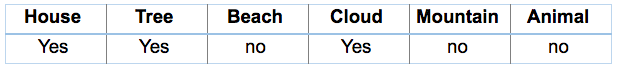These types of problems, where we have a set of target variables, are known as multi-label classification problems. So, is there any difference between these two cases? Clearly, yes because in the second case any image may contain a different set of these multiple labels for different images.

But before going deep into multi-label, I just wanted to clear one thing as many of you might be confused that how this is different from the multi-class problem.

So, let’s us try to understand the difference between these two sets of problems.

## 2. Multi-Label v/s Multi-Class

Consider an example to understand the difference between these two. For this, I hope that below image makes things quite clear. Let’s try to understand it.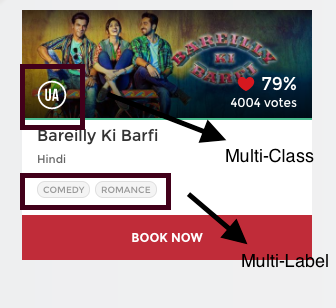For any movie, Central Board of Film Certification, issue a certificate depending on the contents of the movie.

For example, if you look above, this movie has been rated as ‘U/A’ (meaning ‘Parental Guidance for children below the age of 12 years’) certificate. There are other types of certificates classes like ‘A’ (Restricted to adults) or ‘U’ (Unrestricted Public Exhibition), but it is sure that each movie can only be categorized with only one out of those three type of certificates.

In short, there are multiple categories but each instance is assigned only one, therefore such problems are known as multi-class classification problem.

Again, if you look back at the image, this movie has been categorized into comedy and romance genre. But there is a difference that this time each movie could fall into one or more different sets of categories.

Therefore, each instance can be assigned with multiple categories, so these types of problems are known as multi-label classification problem, where we have a set of target labels.

Great! Now you can distinguish between a multi-label and multi-class problem. So, let’s start how to deal with these types of problems.

Scikit-learn has provided a separate library scikit-multilearn for multi label classification.

For better understanding, let us start practicing on a multi-label dataset. You can find a real-world data set from the repository provided by MULAN package. These datasets are present in ARFF format.

So, for getting started with any of these datasets, look at the python code below for loading it onto your jupyter notebook. Here I have downloaded the yeast data set from the repository.

```import scipy
from scipy.io import arff
df = pd.DataFrame(data)```

There is how the data set looks like.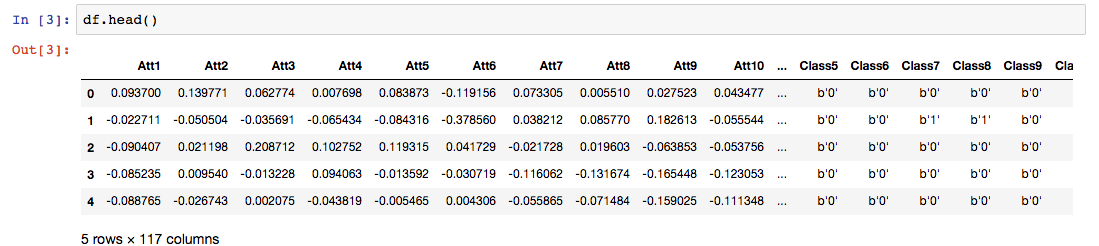Here, Att represents the attributes or the independent variables and Class represents the target variables.

For practice purpose, we have another option to generate an artificial multi-label dataset.

```from sklearn.datasets import make_multilabel_classification

# this will generate a random multi-label dataset
X, y = make_multilabel_classification(sparse = True, n_labels = 20,
return_indicator = 'sparse', allow_unlabeled = False)```

Let us understand the parameters used above.

sparse: If True, returns a sparse matrix, where sparse matrix means a matrix having a large number of zero elements.

n_labels:  The average number of labels for each instance.

return_indicator: If ‘sparse’ return Y in the sparse binary indicator format.

allow_unlabeled: If True, some instances might not belong to any class.

You must have noticed that we have used sparse matrix everywhere, and scikit-multilearn also recommends to use data in the sparse form because it is very rare for a real-world data set to be dense. Generally, the number of labels assigned to each instance is very less.

Okay, now we have our datasets ready so let us quickly learn the techniques to solve a multi-label problem.

## 4. Techniques for Solving a Multi-Label classification problem

Basically, there are three methods to solve a multi-label classification problem, namely:

1. Problem Transformation
3. Ensemble approaches

### 4.1 Problem Transformation

In this method, we will try to transform our multi-label problem into single-label problem(s).

This method can be carried out in three different ways as:

1. Binary Relevance
2. Classifier Chains
3. Label Powerset

#### 4.1.1 Binary Relevance

This is the simplest technique, which basically treats each label as a separate single class classification problem.

For example, let us consider a case as shown below. We have the data set like this, where X is the independent feature and Y’s are the target variable.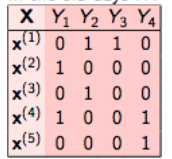In binary relevance, this problem is broken into 4 different single class classification problems as shown in the figure below.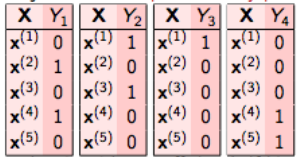We don’t have to do this manually, the multi-learn library provides its implementation in python. So, let’s us quickly look at its implementation on the randomly generated data.

```# using binary relevance
from skmultilearn.problem_transform import BinaryRelevance
from sklearn.naive_bayes import GaussianNB

# initialize binary relevance multi-label classifier
# with a gaussian naive bayes base classifier
classifier = BinaryRelevance(GaussianNB())

# train
classifier.fit(X_train, y_train)

# predict
predictions = classifier.predict(X_test)```

NOTE: Here, we have used Naive Bayes algorithm but you can use any other classification algorithm.

Now, in a multi-label classification problem, we can’t simply use our normal metrics to calculate the accuracy of our predictions. For that purpose, we will use accuracy score metric. This function calculates subset accuracy meaning the predicted set of labels should exactly match with the true set of labels.

So, let us calculate the accuracy of the predictions.

```from sklearn.metrics import accuracy_score
accuracy_score(y_test,predictions)```
`0.45454545454545453`
So, we have attained an accuracy score of 45%, which is not too bad. Let’s us quickly look at its pros and cons.

It is most simple and efficient method but the only drawback of this method is that it doesn’t consider labels correlation because it treats every target variable independently.

#### 4.1.2 Classifier Chains

In this, the first classifier is trained just on the input data and then each next classifier is trained on the input space and all the previous classifiers in the chain.

Let’s try to this understand this by an example. In the dataset given below, we have X as the input space and Y’s as the labels.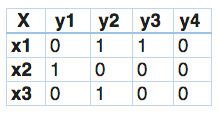In classifier chains, this problem would be transformed into 4 different single label problems, just like shown below. Here yellow colored is the input space and the white part represent the target variable.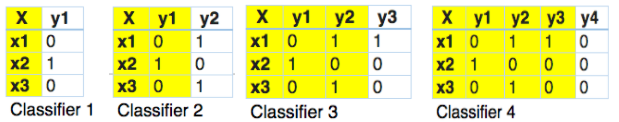This is quite similar to binary relevance, the only difference being it forms chains in order to preserve label correlation. So, let’s try to implement this using multi-learn library.

```# using classifier chains
from skmultilearn.problem_transform import ClassifierChain
from sklearn.naive_bayes import GaussianNB

# initialize classifier chains multi-label classifier
# with a gaussian naive bayes base classifier
classifier = ClassifierChain(GaussianNB())

# train
classifier.fit(X_train, y_train)

# predict
predictions = classifier.predict(X_test)

accuracy_score(y_test,predictions)```
`0.21212121212121213`

We can see that using this we obtained an accuracy of about 21%, which is very less than binary relevance. This is maybe due to the absence of label correlation since we have randomly generated the data.

#### 4.1.3 Label Powerset

In this, we transform the problem into a multi-class problem with one multi-class classifier is trained on all unique label combinations found in the training data.

Let’s understand it by an example.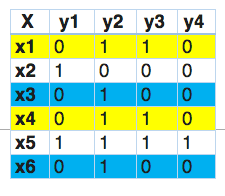In this, we find that x1 and x4 have the same labels, similarly, x3 and x6 have the same set of labels. So, label powerset transforms this problem into a single multi-class problem as shown below.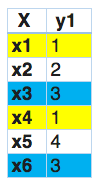So, label powerset has given a unique class to every possible label combination that is present in the training set.

Let’s us look at its implementation in python.

```# using Label Powerset
from skmultilearn.problem_transform import LabelPowerset
from sklearn.naive_bayes import GaussianNB

# initialize Label Powerset multi-label classifier
# with a gaussian naive bayes base classifier
classifier = LabelPowerset(GaussianNB())

# train
classifier.fit(X_train, y_train)

# predict
predictions = classifier.predict(X_test)

accuracy_score(y_test,predictions)```
`0.5757575757575758`

This gives us the highest accuracy among all the three we have discussed till now. The only disadvantage of this is that as the training data increases, number of classes become more. Thus, increasing the model complexity, and would result in a lower accuracy.

Now, let us look at the second method to solve multi-label classification problem.

Adapted algorithm, as the name suggests, adapting the algorithm to directly perform multi-label classification, rather than transforming the problem into different subsets of problems.

For example, multi-label version of kNN is represented by MLkNN. So, let us quickly implement this on our randomly generated data set.

```from skmultilearn.adapt import MLkNN

classifier = MLkNN(k=20)

# train
classifier.fit(X_train, y_train)

# predict
predictions = classifier.predict(X_test)

accuracy_score(y_test,predictions)```
`0.69`

Great! You have achieved an accuracy score of 69% on your test data.

Sci-kit learn provides inbuilt support of multi-label classification in some of the algorithm like Random Forest and Ridge regression. So, you can directly call them and predict the output.

### 4.3 Ensemble Approaches

Ensemble always produces better results. Scikit-Multilearn library provides different ensembling classification functions, which you can use for obtaining better results.

For the direct implementation, you can check out here.

## 5. Case Studies

Multi-label classification problems are very common in the real world. So, let us look at some of the areas where we can find the use of them.

### 1. Audio Categorization

We have already seen songs being classified into different genres. They are also been classified on the basis of emotions or moods like “relaxing-calm”, or “sad-lonely” etc.

### 2. Image Categorization

Multi-label classification using image has also a wide range of applications. Images can be labeled to indicate different objects, people or concepts.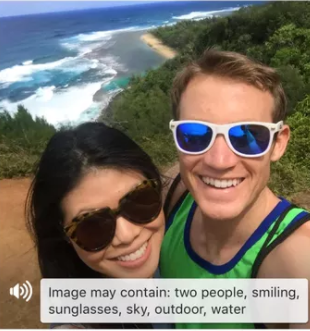### 3. Bioinformatics

Multi-Label classification has a lot of use in the field of bioinformatics, for example, classification of genes in the yeast data set.

It is also used to predict multiple functions of proteins using several unlabeled proteins. You can check this paper for more information.

### 4. Text Categorization

You all must once check out google news. So, what google news does is, it labels every news to one or more categories such that it is displayed under different categories. For example, take a look at the image below.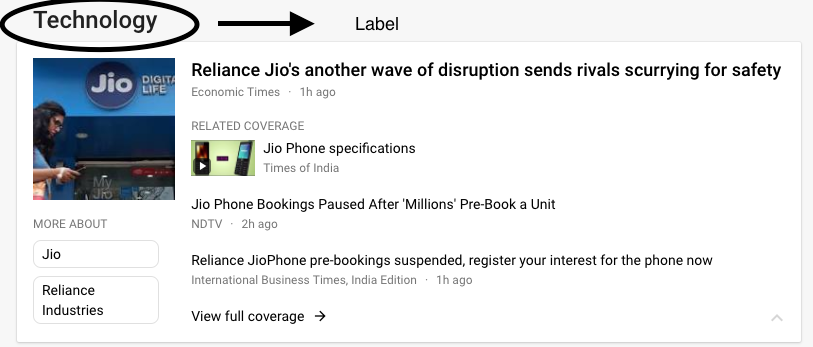That same news is present under the categories of India, Technology, Latest etc. because it has been classified into these different labels. Thus making it a multi label classification problem.

There are plenty of other areas, so explore and comment down below if you wish to share it with the community.

## 6. End Notes

In this article, I introduced you to the concept of multi-label classification problems. I have also covered the approaches to solve this problem and the practical use cases where you may have to handle it using multi-learn library in python.
I hope this article will give you a head start when you face these kinds of problems. If you have any doubts/suggestions, feel free to reach out to me below!

You can also read this article on our Mobile APP•Hemanthkumar E says:

Hi, Shubham great article.
In the label power set method. Once the problem is transformed to single multi class problem you have drawn a table with x and y1 column. I didn’t get how you got the values in y1 column. Can you explain?

Moreover, being a ceramic engineer you have great knowledge in ML. Great!!

•Shubham Jain says:

In label power set, every unique combination of labels has been a separate category. Thus, transforming it into a multi-class problem. For example, in the above problem:
x1 and x4 have the same set of labels, so they are given same class. Further x3 and x6 also have the same set, so they are also given same class. Rest all the other are unique, so they are given different classes.

Cheers
Shubham

•Mahen says:

Dear Shubham,
Can you please tell me the step by step procedure for extracting features from text, extracting features from audio and extracting features from video for sentiment analysis. Its very useful for my research please.

•Sahar says:

Thank you, Shubham, for making this concept so easy for us to understand.

I just have a suggestion: could you please include your code sufficiently so that we could quickly copy/paste it and see the result ? For example, your are using ‘X_train’ and ‘y_train’, but haven’t defined them. Or, some “import”s are missing.
It also took me a while to install skmultilearn. It would have been useful if you would had given a quick hint.

Many thanks once more.

•Shubham Jain says:

Yes, thank you for bringing in the notice. ‘x_train’ and ‘y_train’ are formed by using the train_test_split method on the randomly generated data.

For the installation part, you can simply do ‘pip install scikit-multilearn’ on the terminal.
Can you tell me any other thing that I have missed out and I will definitely take care of this next time.

Cheers!
Shubham

•Navid says:

Hi Shubham. Thanks for your great tutorial. Can you explain a bit in case of text multi-label classification, which transformation function should be used to converts raw input ( string) to test/train data?

Navid

•Gunjan Jhawar says:

•Stéphane says:

Great article and very applied. thanks mate !

•Marco says:

Hi

How can I from

convert the input data into train and test?

Thanks

•Shubham Jain says:

data contains the actual data values which can be further split into train and test data for modelling purposes.

•Marco says:

Can you provide code example?

•Tsu says:

Hi,
Nice tutorial. Do you have any suggestion to get class data and its associated attributes stored in a mysql table into the form as the yeast datasets (atr1 ,atr2 … arN … class1, class2 … class N) ?

•susan abraham says:

Hi ,

•Ellery says:

Hi Shubham Jain

Thanks for this post. I have translated this post to Chinese here: https://github.com/alucard001/Solving-Multi-Label-Classification-problems.

May I ask if I can put it here for reference?

Thank you very much.

•Gopikrishna says:

Hi Shubham Jain,
Thanks for ur great tutorial. 🙂
Can you please explain the same concept on handling multi-target variables on regression problems

•VARUN JAIN says:

Hi Shubham
I have a dataset of multilabel class problem. I want to know the number of classes Label Powerset will make.
Please tell how we can find this.

•Usman Haider says:

Hi Shubham Jain,
Awesome tutorial. I’m working on a multi-label classification model and I’m using Label Power Set. I have used your code but when I call ‘fit()’ method I get following error.
[[[TypeError: no supported conversion for types: (dtype(‘O’),)]]]]
I haven’t used any column with dtype (string/object). All the columns are either category,int,float. Can you help me with this?
My total target labels are Y (1,2,3,4,5,6). Total 6 unique columns

•RAGHAV NYATI says:

Nice Tutorial!

•ashishsinha.iitr says:

Great explanation

•Premal Sheth says:

Hi
Can you please provide dataset because given link for Mulan package is not working it’s showing error: “503 Backand server not available”?

•Aishwarya Singh says: